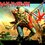# Need help with quicksort

I know this is kind of stupid but I was trying to implement quicksort in Python and it runs into an infinite loop can anyone tell me what's wrong??The partition subroutine works perfectly....The error is in quicksort..Here's the code

  1 2 3 4 5 6 7 8 9 10 11 12 13 14 15  def QuickSort(A,p,r): if p < r: q = Partition(A,p,r) QuickSort(A,0,q) QuickSort(A,q+1,r) def Partition(A, l, r): p = A[l] i = l+1 for j in range(l+1,r): if A[j] < p: A[j],A[i] = A[i],A[j] i += 1 A[l],A[i-1] = A[i-1],A[l] return A.index(p)7 years, 2 months ago

This discussion board is a place to discuss our Daily Challenges and the math and science related to those challenges. Explanations are more than just a solution — they should explain the steps and thinking strategies that you used to obtain the solution. Comments should further the discussion of math and science.

When posting on Brilliant:

• Use the emojis to react to an explanation, whether you're congratulating a job well done , or just really confused .
• Ask specific questions about the challenge or the steps in somebody's explanation. Well-posed questions can add a lot to the discussion, but posting "I don't understand!" doesn't help anyone.
• Try to contribute something new to the discussion, whether it is an extension, generalization or other idea related to the challenge.

MarkdownAppears as
*italics* or _italics_ italics
**bold** or __bold__ bold
- bulleted- list
• bulleted
• list
1. numbered2. list
1. numbered
2. list
Note: you must add a full line of space before and after lists for them to show up correctly
paragraph 1paragraph 2

paragraph 1

paragraph 2

[example link](https://brilliant.org)example link
> This is a quote
This is a quote
    # I indented these lines
# 4 spaces, and now they show
# up as a code block.

print "hello world"
# I indented these lines
# 4 spaces, and now they show
# up as a code block.

print "hello world"
MathAppears as
Remember to wrap math in $$ ... $$ or $ ... $ to ensure proper formatting.
2 \times 3 $2 \times 3$
2^{34} $2^{34}$
a_{i-1} $a_{i-1}$
\frac{2}{3} $\frac{2}{3}$
\sqrt{2} $\sqrt{2}$
\sum_{i=1}^3 $\sum_{i=1}^3$
\sin \theta $\sin \theta$
\boxed{123} $\boxed{123}$

Sort by:

@Thaddeus Abiy @Ammar Fathin Sabili @Trevor B. Please help!!CS is one of the subjects that I study the most but failing such a trivial and known question today is driving me mad!

- 7 years, 2 months ago

- 7 years, 2 months ago

 1 2 3 4 5 6 def Quicksort(A,p,r): if p < r: q = Partition(A,p,r) Quicksort(A,p,q-1) #Mistake is here Quicksort(A,q+1,r) return A 

I am reasonably sure that this is what would work. @josh silverman, can you confirm?

- 7 years, 2 months ago

to start, your Quicksort doesn't return anything

Staff - 7 years, 2 months ago

Fixed!!Thanks a lot.....It feels so frustrated to do stupid mistakes on a known problem............

- 7 years, 2 months ago